# Physics Sample PaperProblem 1.

The location of a solid particle of mass M is determined by the following equations:

X=A+Bt+C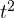Y=D-Et+Fcos(Gt)                 Z=H+Fsin(Gt)

Where A…H are constants.

Determine the three components of the acceleration as a function of time. Does the time have influence on the direction and magnitude of the acceleration?

Solution

The acceleration is the second deliverable of distance. Therefore, three components of the acceleration can be found as follows: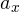(t)=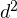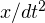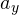(t)=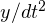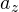(t)=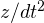Substitution of the equations for X, Y, and Z equations (1) yield:(t)=(A+Bt+C)/d=d(B+2Ct)/dt=2C(t)=(D-Et+Fcos(Gt))/d=d(-E-G*F*sin(Gt))/dt=-F*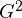*cos(Gt)(t)=(H+Fsin(Gt))/d=d(F*G*cos(Gt))/dt=-F**sin(Gt)

Since at least two of three acceleration components depend on time, an acceleration vector depends on time as well (its magnitude and direction change with time).

Answer:(t)=2C;(t)=-F**cos(Gt);(t)=-F**sin(Gt)

Problem 2.

Three loads exerted on a solid body have the same magnitude. The directions in which the forces act are shown in the picture below. Is it possible for the body to stay at rest? If so, what should be the value of angle Q?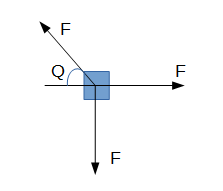Solution

According to Newton’s first law, a body can remain at rest if the sum of all forces acting on this body equals zero. Therefore, the next equation should be true: Σ(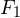+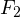+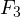)=0. After projecting this equation of equilibrium on the horizontal and vertical axis, we will get:

F*sinQ-F=0

F-F*cosQ=0

Therefore, from the first equation: sin Q=1 and Q=π/2. From the second equation: cos Q=1 and Q=0. Hence, since the angle obtained above are not equal, it is not possible for the body to stay at rest. It would be possible only if the angle between all forces is equal to 2π/3 or if these forces have different magnitudes.

Answer: It is not possible for the body to stay at rest.

Problem 3.

The body undergoes four different loads, as shown in the picture below. Which of the following relations are true in order for the body remain at rest: F=f and N=W; F=f and N>W; F>f and N<W; F>f and N=W?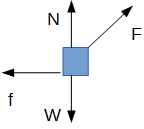Solution

An equilibrium equation should be true for the body: ΣF=0.

After the projection of the forces on the X and Y axes (see the picture below) we will get: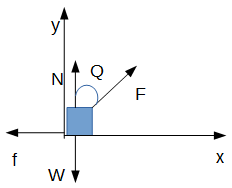F*sinQ-f=0, therefore f=F*sinQ and since sinQ is always <1, fN

Therefore, the following relation is true: F>f and N<W.